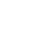# Build and simulate full adder circuit using half adder as a component in VHDL

Code:

```library IEEE;
Use IEEE. STD_LOGIC_1164.all;
port (a,b,cin :in STD_LOGIC;
sum,carry : out STD_LOGIC);
port (p,q :in STD_LOGIC;
s,cy: out STD_LOGIC);
end component;
---------------------------------------or gate component
component or_gate is
port (p1,q1 :in STD_LOGIC;
r1: out STD_LOGIC);
end component;
-------------------------------
signal s1,c1,c2 : STD_LOGIC;
begin
w3: or_gate port map (c1,c2,carry);
end FA;
port (p,q : in STD_LOGIC;
s,cy : out STD_LOGIC);
begin
s <= p xor q;
cy <= p and q;
end HA;
--------------------------------------------or gate program
entity or_gate is
port (p1,q1:in STD_LOGIC;
r1: out STD_LOGIC);
end or_gate;
architecture or_g of or_gate IS
begin
r1 <= p1 or q1;
end or_g;
```

Simulation waveforms:

### 9 thoughts on “Build and simulate full adder circuit using half adder as a component in VHDL”

1.Wow! This blog looks just like my old one! It’s on a entirely different topic but it has pretty much the same layout and design. Superb choice of colors!|

2.thank you

3.Thank You

4.Thank You

5.Thank You

6.Thank You

7.Thank You

8.9.